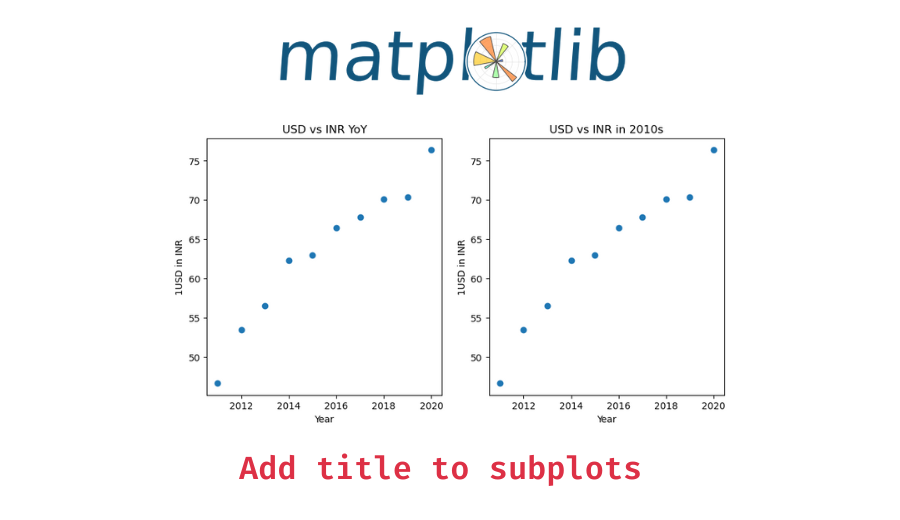# Add Title to Each Subplot in Matplotlib

In this tutorial, we will look at how to add a different title to each subplot in matplotlib with the help of some examples.

When you’re working with a single plot, you can use the `matplot.pyplot` object’s `title()` method to set its title. To set the titles for subplots, however, you have to use the respective subplot’s axes object’s `set_title()` method. Pass the title as an argument.

The following is the syntax –

```# create a plot with two subplots
fig, (ax1, ax2) = plt.subplots(1, 2)

ax1.set_title("Title of subplot1")

ax2.set_title("Title of subplot2")```

Let’s now look at some examples of using the above syntax. First, we will a plot with two subplots without any titles.

```import matplotlib.pyplot as plt

# x values - years
x = [2011, 2012, 2013, 2014, 2015, 2016, 2017, 2018, 2019, 2020]
# y values - 1 USD in INR
y = [46.67, 53.44, 56.57, 62.33, 62.97, 66.46, 67.79, 70.09, 70.39, 76.38]

# create subplots
fig, (ax1, ax2) = plt.subplots(1, 2, figsize=(10, 5))

# plot the first subplot
ax1.scatter(x, y)
ax1.set_xlabel('Year')
ax1.set_ylabel('1USD in INR')

# plot the second subplot
ax2.scatter(x, y)
ax2.set_xlabel('Year')
ax2.set_ylabel('1USD in INR')```

Output:

## Example 1 – Add a different title to each subplot

Here, we plotted the same scatter plot in both subplots but that is not important. Let’s see how we can separately set the title for each subplot.

```# create subplots
fig, (ax1, ax2) = plt.subplots(1, 2, figsize=(10, 5))

# plot the first subplot
ax1.scatter(x, y)
ax1.set_xlabel('Year')
ax1.set_ylabel('1USD in INR')
ax1.set_title('USD vs INR YoY')

# plot the second subplot
ax2.scatter(x, y)
ax2.set_xlabel('Year')
ax2.set_ylabel('1USD in INR')
ax2.set_title('USD vs INR in 2010s')```

Output:

Each subplot now has its own title.

📚 Data Science Programs By Skill Level

Introductory

Intermediate ⭐⭐⭐

🔎 Find Data Science Programs 👨‍💻 111,889 already enrolled

Disclaimer: Data Science Parichay is reader supported. When you purchase a course through a link on this site, we may earn a small commission at no additional cost to you. Earned commissions help support this website and its team of writers.

## Example 2 – Add a title to the entire figure

In case you want to set the title of the entire figure, you can use the `fig` object’s `suptitle()` method. Let’s add an overall title to the above grid of subplots.

```# create subplots
fig, (ax1, ax2) = plt.subplots(1, 2, figsize=(10, 5))

# plot the first subplot
ax1.scatter(x, y)
ax1.set_xlabel('Year')
ax1.set_ylabel('1USD in INR')
ax1.set_title('USD vs INR YoY')

# plot the second subplot
ax2.scatter(x, y)
ax2.set_xlabel('Year')
ax2.set_ylabel('1USD in INR')
ax2.set_title('USD vs INR in 2010s')

fig.suptitle("Foregin currency performance against USD", fontsize=18)```

Output:

We added a title to the entire figure. Note that we additionally passed the font size we want the title to have with the `fontsize` parameter.

You might also be interested in –

•